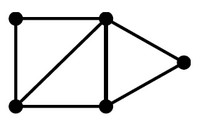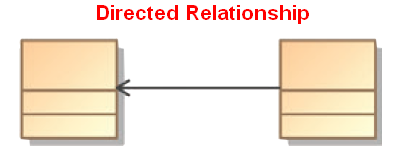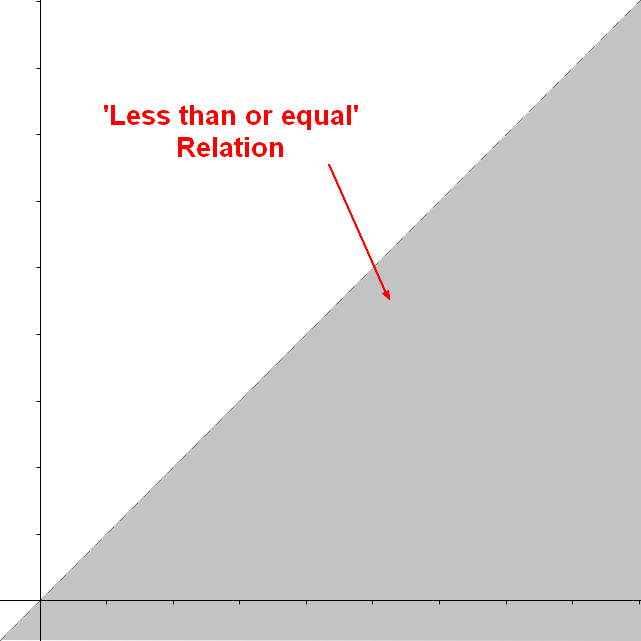# Logical Data Modeling - Symmetric relationship (bi-directional)

A symmetric relation is a type of binary relation that happens when for all a and b in X

• if a is related to b,
• then b is related to a

In mathematical notation, the relation f between x and y is symmetric when $$y = f(x) \\ x= f(y) \\$$

A relation which is not symmetric is asymmetric.

A symmetric relation is an undirected relationship

A symmetric relation is also known as a bi-directional relation.

## Example

• coauthored a paper with,
• distance between
• is married to
• is co-worker of
• is teammate of

## Representation

### Entity Model

When there is no direction on the association, the relationship is symmetric.

### Set

A symmetric relation in the set of {1,2} would be the set of tuple

<1,2>
<2,1>
<1,1> // reflexive
<2,2> // reflexive



### Visual

The relation between x and y is represented by the gray area. The relation is symmetric when $$y = f(x) \\ x= f(y) \\ b = f(a) \\ a = f(b) \\$$

• Symmetric and reflexive (because the relation y=x (the dotted line) is in the relation)

## Documentation / Reference

Discover More(Tree|Nested Set|Hierarchy) Data Structure

A tree is a node that may have children. Tree's are inherently recursive by definition as each child of a node is a Tree itself, with or without children nodes. A tree is a special case of a graph structure...Graph - Direction

A direction is a property of an edge. Directed edges are suitable for modeling asymmetric relations Undirected edges are suitable for modeling symmetric relations An edge is directed if it has a...Graph - Undirected graph

A undirected graph is a graph without direction information Undirected edges are suitable for modeling symmetric relations directed graph
Javascript - Set

The Set interface represents a set data type and works only with primitive type as there is no way to define an object equality function. object Jsdoc Declaration Add/Delete Size Contains...Logical Data Modeling - Antisymmetry relationship

A Antisymmetric relation is a relationship that happens when for all a and b in X: if a is related to b then b isNOT related to a or b=a (reflexivity is allowed) In mathematical notation, an Antisymmetric...Logical Data Modeling - Asymmetric Relation (Uni-directional|Antisymmetric)

An asymmetric relation is a type of binary relation that requiers: antisymmetry (ie if a is related to b, b is not related to a) and irreflexivity (ie an element cannot be related to itself) irreflexivity...Logical Data Modeling - Binary Relation

A binary relation is a relationship between two elements that is implemented via a binary function. Binary relations are used in many branches of mathematics to model concepts like: order relation...Logical Data Modeling - Equivalence Relationship (Equality)

The equivalence relation is a relationship between two entities. The equivalence relation is also known as: is equal to. equality is parallel to (for affine spaces) is in bijection with - bijection...Logical Data Modeling - Reflexive relationship property

reflexive is a relationship property that indicates: that the relationship relates every element to itself. in other word, that the relation set representing the relationship contains every tuple:...Logical Data Modeling - Relationship

A relationship is a model element that relates two entities . In its simple form (semantic form), it's a description that has a verb such as: has consists of uses ... entityentity setrelationship...